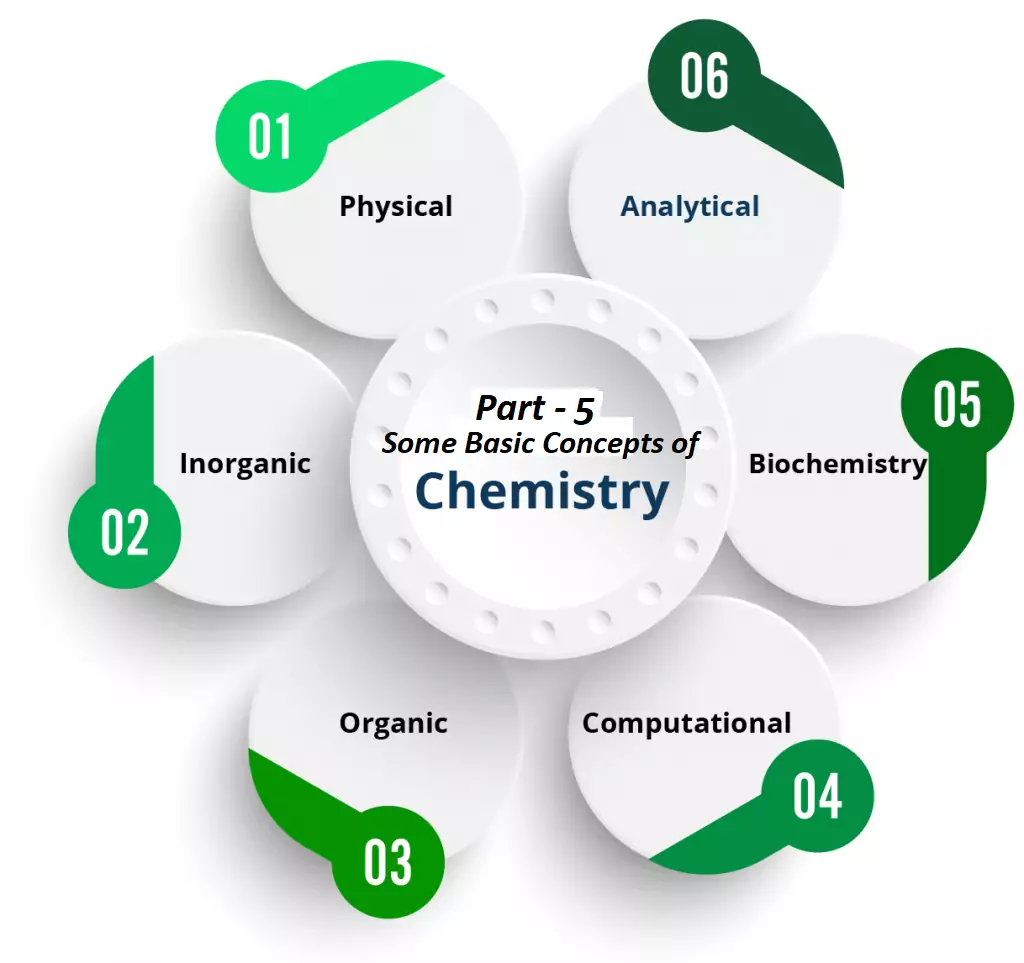# Expressing Concentration Terms with FormulaExpressing Concentration Terms with Formula

Concentration Can be Expressed in the Following Ways:

(1) Mass percent (w/w or m/m): It is defined as the number of parts solute present in 100 parts by mass of solution. i.e.
Mass % of a component =   Mass of solute  × 100
Mass of solution

(2) Molarity (M): It is defined as the number of moles of solute dissolved per liter of solution. i.e.
Molarity (M) = Number of moles of solute (n)/The volume of solution in litre (V)
1 M NaOH solution means 1 mole of NaOH is present in 1 L of solution.
Molarity equation: M1V1   =    M2V2
(Before dilution) =    (After Dilution)
The molarity of a solution decreases in increasing temperature. The molarity of pure water is 55.56 mol L-1.

(3) Molality (m) = It is number of moles of solute dissolved per 1000g (1kg) of solvent.
Molality =  Number of moles of solute/Mass of solvent in kg
Among the above concentration terms, Molarity depends on temperature because it is related to volume, which changes with temperature. Whereas Molality is Independent of Temperature.

Expressing Concentration Terms with Formula

(4) Mole Fraction (x): It is defined as the ratio of the number of moles of a particular component to the total number of moles of solution. i.e.
Mole fraction of a component =  Number of moles of the component/Total number of moles of all the components
Let us consider we have two components 1 and 2. Therefore mole fraction of component 1 is x1, and component 2 is x2.
x1 + x2 = 1 i.e the sum of the mole fractions of all the components in a solution is always equal to 1.

Expressing Concentration Terms with Formula

Numerical Problems

Que 1. According to nutritional guidelines from the US Department of Agriculture, the estimated average requirement for dietary potassium is 4.7 g. What is the estimated average requirement of potassium in moles?
Ans 1. We Know
Number of Moles = Given Mass/ Molecular Mass
Number of moles of K = 4.7/39 = 0.12 mol
Que 2. A litre of air contains 9.2 × 10-4 mol argon. What is the mass of Ar in a litre of air?
Ans 2. We Know
Number of Moles = Given Mass/ Molecular Mass
9.2 × 10-4 = Mass of Argon (Given Mass) / 40
Mass of Argon (Given Mass) = 9.2 × 10-4 × 40 = 0.037 g
Que 3.  Copper is commonly used to fabricate electrical wire.  How many copper atoms are in 5.00 g of copper wire?
Ans 3. We Know
Number of Atoms/Molecules = Number of Moles × Avogadro’s No.
No. of moles of Cu = 5/63.5 = 0.079 mol
Number of Atoms = 0.079 × 6.022 × 1023
= 4.74 × 1022 atoms of Cu
Que 4. A packet of an artificial sweetener contains 40.0 mg of saccharin (C7H5NO3S).  Given that saccharin has a molar mass of 183.18 g/mol, how many saccharin molecules are in a 40.0 mg (0.0400g) sample of saccharin? How many carbon atoms are in the same sample?
Ans 4.  We Know
Number of Atoms/Molecules = Number of Moles × Avogadro’s No.
No of Moles of Saccharin = 0.040/183.18 = 0.000218 mol
Number of Molecules = 0.000218 × 6.022 × 1023 = 0.00131 × 1023
= 1.3 × 1020 Molecules
The compound’s formula (C7H5NO3S) shows that each molecule contains 7 carbon atoms, so the number of C atoms in the given sample is    7 × 1.3 × 1020 = 9.1 × 1020 atoms.

Expressing Concentration Terms with Formula